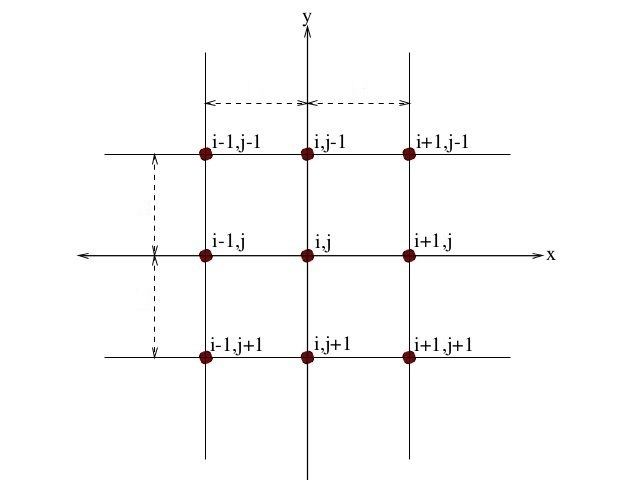# Higher order compact finite difference scheme

Updated onHigh-order compact finite difference schemes are used for solving third-order differential equations created during the study of obstacle boundary value problems. They have been shown to be highly accurate and efficient. They are constructed by modifying the second-order scheme that was developed by Noor and Al-Said in 2002. The convergence rate of the high-order compact scheme is third order, the second-order scheme is fourth order.

Differential equations are essential tools in mathematical modelling. Most physical systems are described in terms of mathematical models that include convective and diffusive transport of some variables. Finite difference methods are amongst the most popular methods that have been applied most frequently in solving such differential equations. A finite difference scheme is compact in the sense that the discretised formula comprises at most nine point stencils which includes a node in the middle about which differences are taken. In addition, greater order of accuracy (more than two) justifies the terminology 'higher-order compact finite difference scheme' (HOC). This can be achieved in several ways. The higher-order compact scheme considered here is by using the original differential equation to substitute for the leading truncation error terms in the finite difference equation. Overall, the scheme is found to be robust, efficient and accurate for most computational fluid dynamics (CFD) applications discussed here further.

The simplest problem for the validation of the numerical algorithms is the Lid Driven cavity problem. Computed results in form of tables, graphs and figures for a fluid with Prandtl number = 0.71 with Rayleigh number (Ra) ranging from 103 to 107 are available in the literature. The efficacy of the scheme is proved when it very clearly captures the secondary and tertiary vortices at the sides of the cavity at high values of Ra.

Another milestone was the development of these schemes for solving two dimensional steady/unsteady convection diffusion equations. A comprehensive study of flow past an impulsively started circular cylinder was made. The problem of flow past a circular cylinder has continued to generate tremendous interest amongst researchers working in CFD mainly because it displays almost all the fluid mechanical phenomena for incompressible, viscous flows in the simplest of geometrical settings. It was able to analyze and visualize the flow patterns more accurately for Reynold's number (Re) ranging from 10 to 9500 compared to the existing numerical results. This was followed by its extension to rotating counterpart of the cylinder surface for Re ranging from 200 to 1000. More complex phenomenon that involves a circular cylinder undergoing rotational oscillations while translating in a fluid is studied for Re as high as 500.

Another benchmark in the history is its extension to multiphase flow phenomena. Natural processes such as gas bubble in oil, ice melting, wet steam are observed everywhere in nature. Such processes also play an important role with the practical applications in the area of biology, medicine, environmental remediation. The scheme has been successively implemented to solve one and two dimensional elliptic and parabolic equation with discontinuous coefficients and singular source terms. These type of problems hold importance numerically because they usually lead to non-smooth or discontinuous solutions across the interfaces. Expansion of this idea from fixed to moving interfaces with both regular and irregular geometries is currently going on.

## References

Similar Topics
Kaaryam Nissaaram
Rohat Alakom
Jordi Sabatés
Topics